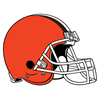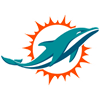DraftKings AFC Championship Odds
+275
2.75 to 1Bills
22.3% implied probability

+600
6 to 1Chiefs
12.0% implied probability

+750
7.5 to 1Chargers
9.9% implied probability

+850
8.5 to 1Broncos
8.8% implied probability

+900
9 to 1Ravens
8.4% implied probability

+1100
11 to 1Bengals
7.0% implied probability

+1200
12 to 1Colts
6.4% implied probability

+1600
16 to 1Browns
4.9% implied probability

+2000
20 to 1Raiders
4.0% implied probability

+2000
20 to 1Dolphins
4.0% implied probability

+2000
20 to 1Titans
4.0% implied probability

+2200
22 to 1Patriots
3.6% implied probability

+5000
50 to 1Steelers
1.6% implied probability

+6500
65 to 1Jaguars
1.3% implied probability

+7500
75 to 1Jets
1.1% implied probability

+13000
130 to 1Texans
0.6% implied probability